# Offset for virtual ground

#### beedees

Joined Mar 29, 2015
37
Hi!
I'm working on a 120 ohm load cell. I decided to use an INA125 to power the bridge (3.4V circa) and to amplify output signal. The full-scale bridge output is +/- 1.06 mV and the initial offset can be in the +/- 1.94 mV range. In order to compensate this initial offset (due to tolerances and temperature) I decided to use a potentiometer in parallel to the bridge. Because the output of the bridge can be positive or negative, the output is referenced to midsupply (3.4/2=1.7 V). (My ADC range is 0-3.6V)
My issue is: how can use INA125 IAref terminal to obtain 1.7 V offset? First I have thought to operate with a voltage divider and a buffer which output is connected to IAref, but resistors must be very accurate i.e low tolerance and TCR. (In my application I have to pay attention to thermal effect).
Another solution I have thought is to use the potentiometer of the bridge in order to obtain an initial 1.7 V offset. In other words, instead of nulling the bridge I can move the wiper until the output is 1.7 V, but I don't know if this method produces a bigger non-linearity.
What do you think about? What is the best solution? Have you other ideas?
Thanks!

#### beedees

Joined Mar 29, 2015
37
This is my idea: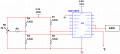By using the potentiometer I can unbalance the bridge. So instead of nulling the output I generate a virtual ground (pseudoground) of 3.3/2=1.65V.
Initially I thought this configuration: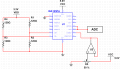but I realized that in this way maybe I can't compensate a possible offset of the bridge. For example, if the initial bridge offset (due to tolerances) is over 1.65V I can't add a negative voltage to obtain 1.65V! So I have decided to try the first configuration.
Are you according to me? Are there errors I'm neglecting?

Joined Jan 15, 2015
7,217
The attached circuit using the INA125 was actually done by another forum member Eric Gibbs. You may want to think about modifying it for your application. Personally I would likely use the INA125 to provide the bridge excitation and get more sensitivity out of the bridge. This would also afford you very good stability for excitation voltage which is real important in the bridge. Would this be a standard load cell of a roll your own version?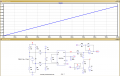Ron

#### beedees

Joined Mar 29, 2015
37
The attached circuit using the INA125 was actually done by another forum member Eric Gibbs.
Yes I have seen it. But I don't understand something. Why do you use a differential amplifier at the output of the INA? In this case you get negative voltages, right?

Personally I would likely use the INA125 to provide the bridge excitation and get more sensitivity out of the bridge. This would also afford you very good stability for excitation voltage which is real important in the bridge.
Yes! I didn't draw in the attached circuits but I use INA125 for this reason.

Would this be a standard load cell of a roll your own version?
It is a 120 load cell. Precisely I haven't to measure a weight but strain. Two of bridge resistances are strain gauges so the output voltage of the bridge can be positive or negative and for this reason I would like to create a middle reference voltage: 3.3/2=1.65. This represent the zero of the bridge i.e. when no load is applied.

Joined Jan 15, 2015
7,217
Yes I have seen it. But I don't understand something. Why do you use a differential amplifier at the output of the INA? In this case you get negative voltages, right?

What he is doing is creating an offset, note in the drawing where V2 comes from.

Yes! I didn't draw in the attached circuits but I use INA125 for this reason.

It is a 120 load cell. Precisely I haven't to measure a weight but strain. Two of bridge resistances are strain gauges so the output voltage of the bridge can be positive or negative and for this reason I would like to create a middle reference voltage: 3.3/2=1.65. This represent the zero of the bridge i.e. when no load is applied.

OK, if you look at this data sheet for the INA125 take a look at Figure 2 Page 11.

#### beedees

Joined Mar 29, 2015
37
Yes, this is the best way certainly. However I decided to use INA125 to avoid generating a precision voltage reference. If I use the configuration of Figure 2 Page 11 I won't be able to avoid this problem, right?
My doubt is: instead of generating an offset using IAref pin, can I unbalance the bridge with the potentiometer I described in post #2 ? So first I unbalance the bridge with 1.65V, and then when the strain is applied the value I will read is over or above 1.65V.
I don't know if this way could be a possible solution... what do you think?
I think the output is nonlinear and I could lose sensitivity.

Joined Jan 15, 2015
7,217
You can't just place a pot on the bridge or it will drift all over the place.

Yes, this is the best way certainly. However I decided to use INA125 to avoid generating a precision voltage reference. If I use the configuration of Figure 2 Page 11 I won't be able to avoid this problem, right?
I have No idea what you are talking about. I would use the available precision voltages available from the INA125 as my bridge excitation. That is what it is there for. Take what you expect the max voltage from the bridge to be and figure out how much to amplify it using the INA125 (Scale the gain of the INA125 to your bridge and desired output). If you are worried about the Zero then use the pin 5 offset capability of the INA125. If you want to run the INA125 off a single supply they explain that also in the data sheet. If you are worried about a zero offset using a single supply then buffer and offset the output. This all should be relatively simple as the IA125 was designed for just this purpose.

Ron

#### beedees

Joined Mar 29, 2015
37
I have No idea what you are talking about. I would use the available precision voltages available from the INA125 as my bridge excitation. That is what it is there for.
Sorry for my insistence but maybe I could not explain very well. I use Vref_out (pin4) as my bridge excitation. Suppose I excite the bridge with 2.5V as you can see in Figure 6 Page 13, exactly in the same manner. In this figure IAref pin (pin 5) is used to increase output (pin 10) of 2.5V (because IAref pin is connected to pin 14).
But I would prefer not use IAref pin. If it is possible I prefer first unbalance the bridge (with potentiometer) up to 2.5 V and then when a load is applied bridge's output will be over or above 2.5 Volt. Sorry for my English, maybe I cannot find the right words...

This was an example. In my application bridge excitation is 3.3 V and I can get it with INA125 connecting opportunely some pins.
To be more clear, now not consider INA, but only the bridge.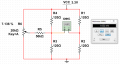Moving the wiper of potentiometer I unbalance the bridge up to 3.3/2=1.65 mV and if the gain is 1000 then output will be 1.65V.
Now if strain gauge is stressed, for example R3, output will be over 1.65 mV.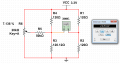and in the same manner if R3<120 then output will be above 1.65 mV.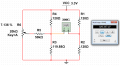In practice my idea is to use an unbalanced bridge, so that I obtain an initial offset. What do you think?
I repeat, sorry for my insistence and my EnglishJoined Jan 15, 2015
7,217
Now I understand what you are saying but... There is no 1.65 Volts. You are taking the 3.3 volt excitation and doing 3.3 / 2 = 1.65 but in a bridge it does not work that way. Yes, if I measure the junction of R1 & R2 with a ground reference I will get 1.65 volts and if I measure the junction of R3 & R4 I will get 1.65 Volts. Those two points are going where? They are going into the + and - inputs of an Instrumentation Amplifier. So what is the difference between 1.65 and 1.65? The difference is Zero because the bridge is Balanced. While either point to ground is 1.65 volts the difference between the two points is Zero and the IA is seeing a Zero and will output a Zero because any gain times zero is zero.

OK, R1, R3 and R4 are precision 120 Ohm 1% resistors with excellent temperature coefficients, R2 is a 120 Ohm strain gauge. With zero strain applied R2 is 120 Ohms and again the bridge is balanced. However, if I apply a strain to the bridge R2 will either increase or decrease in value and the bridge will become unbalanced. That slight unbalance will then be amplified by the IA. That is how it works. The IA is not looking at a voltage to ground, the IA sees two inputs and amplifies the difference.

Normally the bridge output has a sensitivity specification, for example it can look like this: Sensitivity 2mV/V and that means if I have a load cell (compression, expansion or both) that is rated as full scale is 10 Kg then the full scale output of the bridge is 2mV / Volt meaning two milli-volts per volt of excitation. So in this case with 10 Volts excitation my bridge out with a 10Kg load would be 20 mV. If I amplify that times 100 I get a full scale of 2 Volts or 0.2 Volt per Kg.

In conclusion, for your purposes in the measurment plane there is no 1.65 volts to offset.

Ron